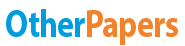# The Multiplicative Model of Time Series Analysis

Essay by   •  June 4, 2019  •  Course Note  •  661 Words (3 Pages)  •  107 Views

## Essay Preview: The Multiplicative Model of Time Series Analysis

Report this essay
Page 1 of 3

The multiplicative model of time series analysis can be expressed as Y = T x S x R

In this model, only T is expressed in the original units, S and R are stated in terms of percentages.

If the random component R is negligible, which means R = 1 in this case, the seasonal percentage is calculated by S = Y/ T

For multiplicative model, the sum of average seasonal factor is the number of values, so 5 in this case. If not, make adjustment.

Forecasting by extrapolation

Extrapolation is the process of estimating, beyond the original observation range, the value of a variable on the basis of its relationship with another variable.

Use the trend T and seasonal factor S to forecast the unknown Y values in a near future.

In this examples, we can predict the output in each day of the forth week.

To have the Y values for each day of week 4, we need both trend values T and seasonal values S. For S: use adjusted average S for each day.

For T: we need to estimate it from known T-values. Then for additive model Y = T+S

multiplicative model Y = T x S

Trend T in this example is in an increasing trend. Then we calculate the average increase of T over each time period. Min value = 103.2, max value = 110.2. 11 boundary points => 10 periods.

Average increase of T = (110.2 – 103.2) / 10 = 0.7

Hence, for he unknown T-values, we add 0.7 to the previous value to get the next value.

We make time period Mon-Fri to week 4

Now, to forecast the Y-values for additive model: Y=T+S

Multiplicative Y = TxS

Mon: 100                                         99

Tue: 129                                         130

Wed: 114                                        114

Thu: 142                                        144

Fri: 84                                                81

There is a slight difference between two models. Which model is more reliable?

• Both models are reliable

...

...